### Abstract

Neural Radiance Fields (NeRFs) have shown promise in applications like view synthesis and depth estimation, but their learning from multiview images faces inherent uncertainties. Current methods to quantify them are either heuristic or computationally demanding. We introduce BaysRays, a post-hoc framework to evaluate uncertainty in any pre-trained NeRF without modifying the training process. Our method establishes a volumetric uncertainty field using spatial perturbations and a Bayesian Laplace approximation. We derive our algorithm statistically and highlight its superior performance in key metrics and NeRF-related applications.

### Epistemic Uncertainty in NeRF: What does it mean intuitively?

NeRFs rely on training images, taken as 2D projections of a 3D scene, to reconstruct the scene geometry and appearance. Inherently, the projection causes loss of information and leads to ambiguity in the reconstruction problem, making multiple solutions valid for the reconstruction. This is even more prominent in real scenarios where limited training views of the scene is available. Below is a toy 2D example of a NeRF, where single ray training cameras are observing the scene and the ray color is calculated through volume rendering. All camera rays either start from the top side and end at the bottom or vice versa. Training multiple NeRFs on this training data, leads to different reconstructions, all valid and minimizing the training loss. Analyzing the geometry of this problem, we can see there exist a null space for each of the blue and red segments. One can perturb the learned lines, inside this space freely without hurting the reconstruction problem.

### How to parametrize perturbations?

To parametrize possible perturbations on a pre-trained NeRF (with optimized parameters φ∗) and then analyze if in fact a part of the reconstructed NeRF can be perturbed, we introduce a spatial deformation layer (parametrized by θ) on the input coordinates of NeRf, formalized as below: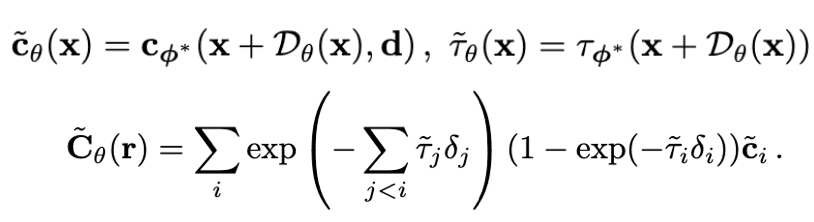where deformation layer is modeled as a tri-linearly interpolatable grid. We assume a likelihood of the same form as with the NeRF parametrization,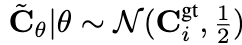. To ensure small perturbations, we place a regularizing independent Gaussian prior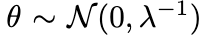on our new parameters: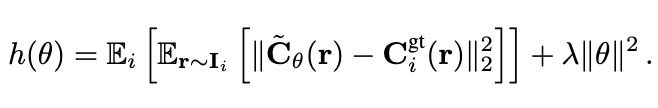It is now possible to minimize the negative log likelihood term above wrt to θ and with φ∗ frozen, to converge to one of the many valid solutions for the spatial perturbations on a given NeRF that do not affect reconstruction loss. The more freely a perturbation at a point can move the point (i.e bigger variance in the perturbation), the more uncertain we are about the true position of that reconstructed point in the space.

### But wait ...

As we already have a fully converged NeRF, we know θ* = 0 is a local minimum for the negative log likelihood loss posed above. Knowing that, we can utilize Laplace Approximation at the local minima of a negative log likelihood function! Laplace Approximation states that with the help of a second order Taylor expansion, the probability distribution described by the negative log likelihood function can be approximated with a Gaussian distribution at the point of minimum. The Covariance matrix of this distribution is then equal to inverse negative Hessian of NLL function at the point of minimum. We show that by using Fisher Information, the Hessian of loss for the already-converged NeRF can be readily computed using 1st order gradients. Further, we show that the resulting Hessian is sparse enough to allow for a diagonal approximation, leading to fast and efficient inversion of the Hessian. Finally, the Covariance matrix of the deformation gird is computed as: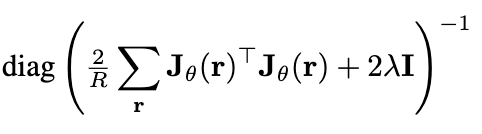The diagonal entries of the Covariance matrix, define a marginal variance vector σ = (σx, σy , σz ) at each grid vertex. The norm of this vector ∥σ ∥ is then a positive scalar that measures the local spatial uncertainty of the radiance field at each grid vertex. Through it, we can define our spatial uncertainty field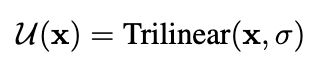### Results

Uncertainty Quantification results: We show correlation of uncertainty to absolute error of given NeRF in depth. Lower the area under the sparsification plot shows higher correlation. Please use the buttons for comparison of pixel ranks in sparsification process, between methods.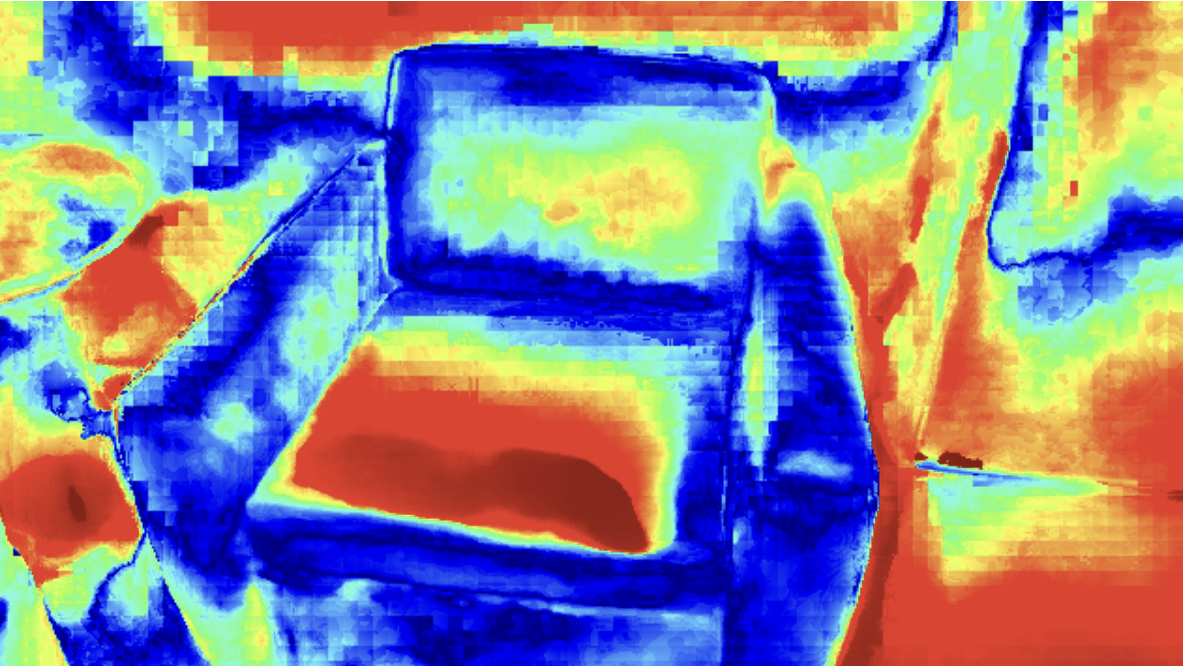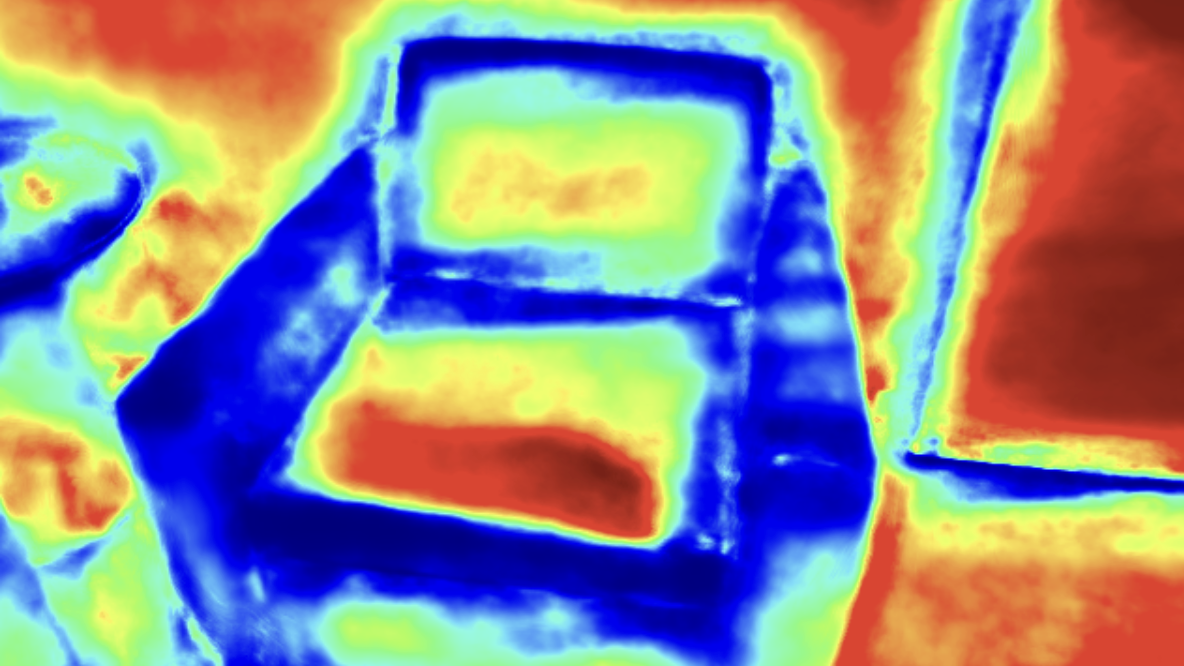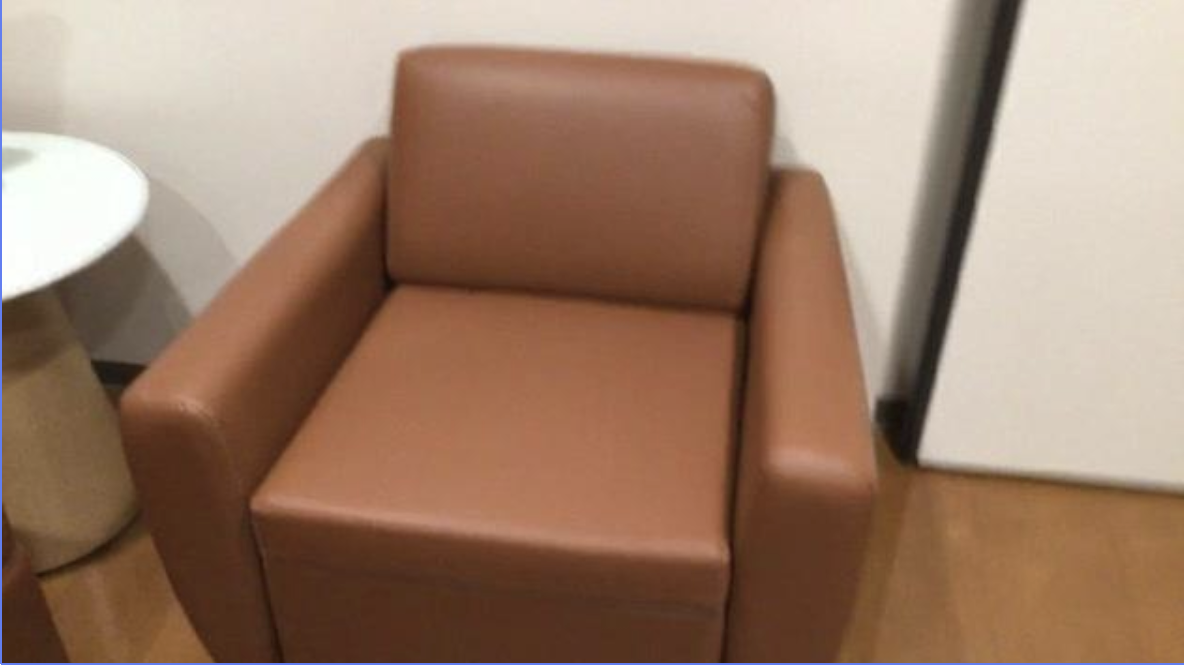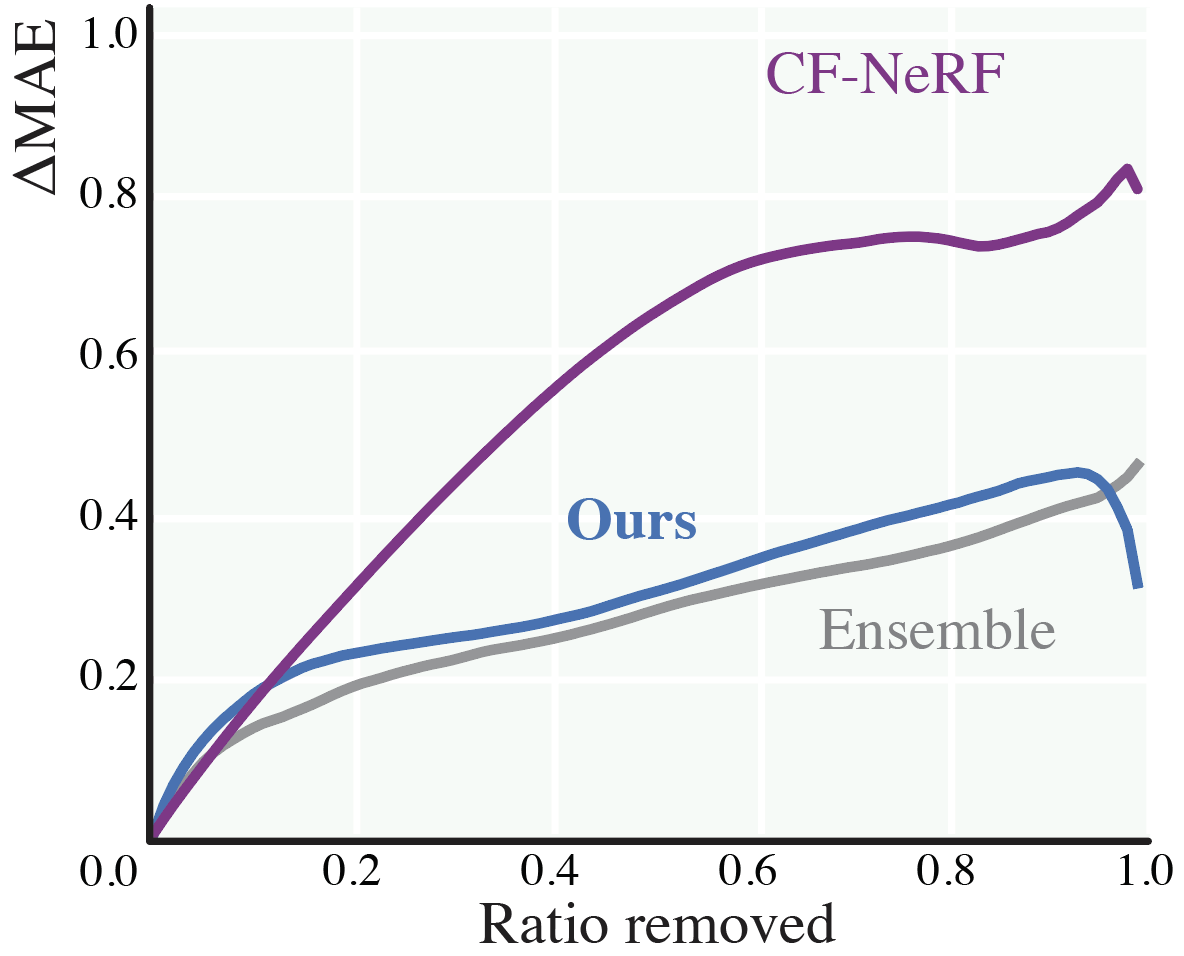Clean-up results:
 Picnic Pikachu Century

Demo:

### Acknowledgements

The website template was borrowed from Dor Verbin.